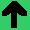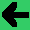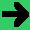# Derived Knowledge

• In Rule based systems, derived knowledge is knowledge that the user neither puts directly into the system, or recieves from the system, but the system uses.
• Pragmatically, a WM item that is on the then side of one rule and the if side of another is derived.
• Why is derived knowledge important? It shows that the system is doing more reasoning. It shows that it is a deeper system.
• What is an example of derived knowledge?
• In noughts and crosses, knowledge that the opponent has two in a row is derived from the board.
• In the second lab, if A then B, if B then C, B is derived knowledge.
• What would be an example of derived knowledge.
• In class work: write a rule that determines that X's have two in a row. Write a rule that uses that fact.